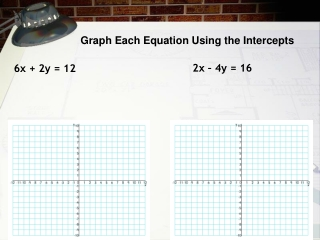Download Presentation6x + 2y = 12

# 6x + 2y = 12

Télécharger la présentation## 6x + 2y = 12

- - - - - - - - - - - - - - - - - - - - - - - - - - - E N D - - - - - - - - - - - - - - - - - - - - - - - - - - -
##### Presentation Transcript

1. Graph Each Equation Using the Intercepts 6x + 2y = 12 2x – 4y = 16

2. (y) (x) A rate of change is a ratio that compares the amount of change in a dependent variable to the amount of change in an independent variable. For any two points on a nonvertical line, this ratio is constant. The constant rate of change of a nonvertical line is call the slope of the line.

3. Rise = 3 Run = 9 Rise = –3 Run = –9 Example 1 - Find the slope of the line. Begin at one point and count vertically to find the RISE. Then count horizontally to the second point to find the RUN.

4. Example 2 - Find the slope of the line.

5. If you know 2 different points on a line, you can use the slope formula to find the slope of the line. RISE RUN

6. Example 3 - Find the slope of the line that contains (5, –7) and (6, –4). Substitute (5, –7) for (x1,y1) and (6, –4) for (x2,y2).

7. Example 4 - Find the slope of the line that contains (2, 5) and (8, 1). Substitute (2, 5) for (x1, y1) and (8, 1) for (x2,y2).

8. Example 5 - Find the slope of each line. B. A. The slope is 0. The slope is undefined.

9. Example 6 - Find the slope of the line that contains (–2, –2) and (7, –2). Substitute (–2, –2) for (x1, y1) and (7, –2) for (x2,y2).

10. As shown in the previous examples, slope can be positive, negative, zero, or undefined.

11. Example 7 - Tell whether the slope of each line is positive, negative, zero, or undefined. B. A. The line rises from left to right. The line falls from left to right. The slope is positive. The slope is negative.

12. Example 7 - Tell whether the slope of each line is positive, negative, zero, or undefined. c. d. The line is vertical. The line rises from left to right. The slope is undefined. The slope is positive.

13. Example 8 - The graph shows the average electricity costs in dollars for operating a refrigerator for several months. Find the slope of the line. Then tell what the slope represents. Step 1 Use the slope formula.

14. In this situation, y represents the total cost of electricity and x represents time. So slope represents in units of . Example 8 Continued Step 2 Tell what the slope represents. A slope of 6 means that the cost of electricity to run the refrigerator is increasing (positive change) at a rate of 6 dollars each month.

15. Example 9 - The graph shows the height of a plant over a period of days. Find the slope of the line. Then tell what the slope represents. Step 1 Use the slope formula.

16. In this situation, y represents the height of the plant and x represents time. So slope represents in units of . A slope of means that the height of the plant is increasing (positive change) at a rate of 1 cm every two days. Example 9 Continued Step 2 Tell what the slope represents.

17. Lesson Quiz: Part I Find the slope of each line. 1. 2. undefined

18. Lesson Quiz: Part II 3. Find the slope of the line that contains (5, 3) and (–1, 4). 4. Find the slope. Then tell what the slope represents. 50 A slope of 50 means that the speed of the bus is 50 mi/h.